International
Tables for
Crystallography
Volume C
Mathematical, physical and chemical tables
Edited by E. Prince

International Tables for Crystallography (2006). Vol. C, ch. 9.5, pp. 790-791

## Section 9.5.2. Methodology

F. H. Allen,a D. G. Watson,a L. Brammer,b A. G. Orpenc and R. Taylora

aCambridge Crystallographic Data Centre, 12 Union Road, Cambridge CB2 1EZ, England,bDepartment of Chemistry, University of Missouri–St Louis, 8001 Natural Bridge Road, St Louis, MO 63121-4499, USA, and cSchool of Chemistry, University of Bristol, Bristol BS8 1TS, England

### 9.5.2. Methodology

| top | pdf |

#### 9.5.2.1. Selection of crystallographic data

| top | pdf |

All results given in Table 9.5.1.1are based on X-ray and neutron diffraction results retrieved from the September 1985 version of the CSD. Neutron diffraction data only were used to derive mean bond lengths involving H atoms. This version of the CSD contained results for 49 854 single-crystal diffraction studies of organo-carbon compounds; 10 324 of these satisfied the acceptance criteria listed below and were used in the averaging procedures:

 (i) Structure is `organic', i.e. belongs to the CSD chemical classes 1–65 or 70 (Cambridge Crystallographic Data Centre User Manual, 1978). (ii) Atomic coordinates for the structure have been published and are available in the CSD. (iii) Structure was determined from diffractometer data. (iv) Structure does not contain unresolved numeric data errors from the original publication (such errors are usually typographical and are normally resolved by consultation with the authors). (v) Structure was not reported to be disordered. (vi) Only structures of higher precision were included on the basis of either (a) the crystallographic R factor was0.07 and the reported mean estimated standard deviation (e.s.d.) of the C—C bond lengths was0.010 Å (corresponds to AS flag = 1 or 2 in the CSD), or (b) the crystallographic R factor0.05 and the mean e.s.d. for C—C bonds was not available in the database (AS = 0 in the CSD). (vii) Where the structure of a given compound had been determined more than once within the limits of (i)–(vi), then only the most precise determination was used.

#### 9.5.2.2. Program system

| top | pdf |

All calculations were performed on the University of Cambridge IBM 3081 D using the programs BIBSER, CONNSER, RETRIEVE, GEOM78, and PLUTO78 (Allen et al., 1979). A stand-alone program was written to implement the selection criteria, whilst a new program (STATS) was written to perform the statistical calculations described below. It was also necessary to modify CONNSER to improve the precision with which it locates chemical substructures. In particular, the program was altered to permit the location of atoms with specified coordination numbers. This was essential in the case of carbon so that atoms with coordination numbers 2, 3 and 4 (equivalent to formal hybridization states sp1, sp2, sp3) could be distinguished easily and reliably. Considerable care was taken to ensure that the correct molecular fragment was located by GEOM78 in the generation of geometrical tabulations. This often involved the explicit specification of H atoms in fragments, and the extensive use of geometrical tests on valence and torsion angles. Considerable use was also made of chemical structural diagrams, which are available in the Cambridge in-house version of the CSD for some 81% of all entries. Chemical diagrams proved useful, for example, in identifying the various coordination environments commonly adopted by atoms such as As, B, P, etc.

#### 9.5.2.3. Classification of bonds

| top | pdf |

The classification of bonds used in Table 9.5.1.1is based on common functional groups, rings and ring systems, coordination spheres, etc. It is designed: (i) to appear logical, useful and reasonably self-explanatory to chemists, crystallographers, and others who may use the table; (ii) to permit a meaningful average value to be cited for each bond length. With reference to (ii), it was considered that a sample of bond lengths could be averaged meaningfully if: (a) the sample was unimodally distributed; (b) the sample standard deviation (σ) was reasonably small, ideally less than ca 0.02 Å; (c) there were no conspicuous outlying observations – those that occurred at > 4σ from the mean were automatically eliminated from the sample by STATS, other outliers were inspected carefully; (d) there were no compelling chemical reasons for further subdivision of the sample.

#### 9.5.2.4. Statistics

| top | pdf |

Where there are less than four independent observations of a given bond length, then each individual observation is given explicitly in the table. In all other cases, the following statistics were generated by the program STATS.

 (i) The unweighted sample mean, d, where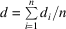andis the ith observation of the bond length in a total sample of n observations. Recent work (Taylor & Kennard, 1983, 1985, 1986) has shown that the unweighted mean is an acceptable (even preferable) alternative to the weighted mean, where the ith observation is assigned a weight equal to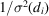. This is especially true (Taylor & Kennard, 1985) where structures have been pre-screened on the basis of precision. (ii) The sample median, m. This has the property that half of the observations in the sample exceed m, and half fall short of it. (iii) The sample standard deviation, denoted here as σ, where: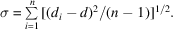(iv) The lower quartile for the sample,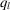. This has the property that 25% of the observations are less thanand 75% exceed it. (v) The upper quartile for the sample,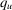. This has the property that 25% of the observations exceed, and 75% fall short of it. (vi) The number (n) of observations in the sample.

The statistics given in the final table correspond to distributions for which the automatic 4σ cut-off (see above) had been applied, and any manual removal of additional outliers (an infrequent operation) has been performed. In practice, a very small percentage of observations was excluded by these methods. The major effect of removing outliers is to improve the sample standard deviation, as shown in Fig. 9.5.2.1in which a single observation is deleted.Figure 9.5.2.1 | top | pdf |Effect of the removal of outliers (contributors that are > 4σ from the mean) for the C—C bond in Car—C≡N fragments. Relevant statistics (see text) are: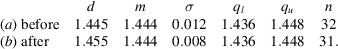The statistics chosen for tabulation effectively describe the distribution of bond lengths in each case. For a symmetrical, normal distribution: the mean (d) will be approximately equal to the median (m); the lower and upper quartiles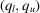will be approximately symmetric about the median: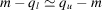, and 95% of the observations may be expected to lie within ±2σ of the mean value. For a skewed distribution, d and m may differ appreciably andandwill be asymmetric with respect to m. When a bond-length distribution is negatively skewed as in Fig. 9.5.2.2, i.e. very short values are more common than very long values, then it may be due to thermal-motion effects; the distances used to prepare the table were not corrected for thermal libration.Figure 9.5.2.2 | top | pdf |Skewed distribution of B—F bond lengths in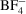ions: d = 1.365, m = 1.372, σ = 0.029, ql = 1.352, qu = 1.390 for 84 observations. Note that d ≠ m and that ql, qu are asymmetrically disposed about the mean d.

In a number of cases, the initial bond-length distribution was clearly bimodal, as in Fig. 9.5.2.3(a). All cases of bimodality were resolved on chemical grounds before inclusion in the table, on the basis of hybridization, conformation-dependent conjugation interactions, etc. For example, the histogram of Fig. 9.5.2.3(a)was resolved into the two discrete unimodal distributions of Figs. 9.5.2.3(b), (c), which correspond to planar N(sp2), pyramidal N(sp3), respectively. The mean valence angle at N was used as the discriminator, with a range of 108–114° for Nsp3 and117.5° for Nsp2.Figure 9.5.2.3 | top | pdf |Resolution of the bimodal distribution of C—N bond lengths in Car —N(Csp3)2 fragments: (a) complete distribution; (b) distribution for planar N, mean valence angle at N > 117.6°; (c) distribution for pyramidal N, mean valence angle at N in the range 108–114°.

### References

Allen, F. H., Bellard, S., Brice, M. D., Cartwright, B. A., Doubleday, A., Higgs, H., Hummelink, T., Hummelink-Peters, B. G., Kennard, O., Motherwell, W. D. S., Rodgers, J. R. & Watson, D. G. (1979). The Cambridge Crystallographic Data Centre: computer-based search, retrieval, analysis and display of information. Acta Cryst. B35, 2331–2339.
Cambridge Crystallographic Data Centre User Manual (1978). 2nd ed. Cambridge University, England.
Taylor, R. & Kennard, O. (1983). The estimation of average molecular dimensions from crystallographic data. Acta Cryst. B39, 517–525.
Taylor, R. & Kennard, O. (1985). The estimation of average molecular dimensions. 2. Hypothesis testing with weighted and unweighted means. Acta Cryst. A41, 85–89.
Taylor, R. & Kennard, O. (1986). Cambridge Crystallographic Data Centre. 7. Estimating average molecular dimensions from the Cambridge Structural Database. J. Chem. Inf. Comput. Sci. 26, 28–32.